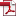• KSII Transactions on Internet and Information Systems
Monthly Online Journal (eISSN: 1976-7277)

# A General Design Method of Constructing Fully Homomorphic Encryption with Ciphertext Matrix

Vol. 13, No. 5, May 30, 201910.3837/tiis.2019.05.022, Download Paper (Free):#### Abstract

It is important to construct fully homomorphic encryption with ciphertext matrix that makes fully homomorphic encryption become very nature and simple. We present a general design method of constructing fully homomorphic encryption whose ciphertext is matrix. By using this design method, we can deduce a fully homomorphic encryption scheme step by step based on a basic encryption scheme. The process of deduction is similar to solving equation and the final output result is a fully homomorphic encryption scheme with ciphertext matrix. The idea of constructing ciphertext matrix is ciphertexts stack, which don’t simply stack ciphertexts together but is to obtain the desired homomorphic property. We use decryption structure as tool to analyze homomorphic property and noise growth during homomorphic evaluation. By using this design method, we obtain three corresponding fully homomorphic encryption schemes. Our obtained fully homomorphic encryption schemes are more efficient. Finally, we introduce the adversary advantage and improve the previous method of estimating concert parameters of fully homomorphic encryption. We give the concert parameters of these schemes.